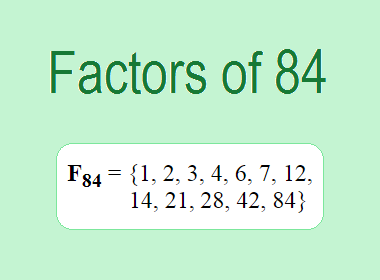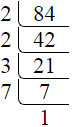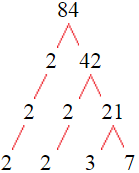# Factors of 84The factors of 84 are 1, 2, 3, 4, 6, 7, 12, 14, 21, 28, 42, and 84 i.e. F84 = {1, 2, 3, 4, 6, 7, 12, 14, 21, 28, 42, 84}. The factors of 84 are those numbers that can divide 84 without leaving a remainder.

We can check if these numbers are factors of 84 by dividing 84 by each of them. If the result is a whole number, then the number is a factor of 84. Let's do this for each of the numbers listed above:

·        1 is a factor of 84 because 84 divided by 1 is 84.

·        2 is a factor of 84 because 84 divided by 2 is 42.

·        3 is a factor of 84 because 84 divided by 3 is 28.

·        4 is a factor of 84 because 84 divided by 4 is 21.

·        6 is a factor of 84 because 84 divided by 6 is 14.

·        7 is a factor of 84 because 84 divided by 7 is 12.

·        12 is a factor of 84 because 84 divided by 12 is 7.

·        14 is a factor of 84 because 84 divided by 14 is 6.

·        21 is a factor of 84 because 84 divided by 21 is 4.

·        28 is a factor of 84 because 84 divided by 28 is 3.

·        42 is a factor of 84 because 84 divided by 42 is 2.

·        84 is a factor of 84 because 84 divided by 84 is 1.

## How to Find Factors of 84?

1 and the number itself are the factors of every number. So, 1 and 84 are two factors of 84. To find the other factors of 84, we can start by dividing 84 by the numbers between 1 and 84. If we divide 84 by 2, we get a remainder of 0. Therefore, 2 is a factor of 84. If we divide 84 by 3, we get a remainder of 0. Therefore, 3 is also a factor of 84.

Next, we can check if 4 is a factor of 84. If we divide 84 by 4, we get a remainder of 0. Therefore, 4 is also a factor of 84. If we divide 84 by 5, we get a remainder of 4. Therefore, 5 is not a factor of 84. We can continue this process for all the possible factors of 84.

Through this process, we can find that the factors of 84 are 1, 2, 3, 4, 6, 7, 12, 14, 21, 28, 42, and 84. These are the only numbers that can divide 84 without leaving a remainder.

********************

********************

## Properties of the Factors of 84

The factors of 84 have some interesting properties. One of the properties is that the sum of the factors of 84 is equal to 224. We can see this by adding all the factors of 84 together:

1 + 2 + 3 + 4 + 6 + 7 + 12 + 14 + 21 + 28 + 42 + 84 = 224

Another property of the factors of 84 is that the prime factors of 84 are 2, 3, and 7 only and there are all composite numbers except 1, 2, 3, and 7.

## Applications of the Factors of 84

The factors of 84 have several applications in mathematics. One of the applications is in finding the highest common factor (HCF) of two or more numbers. The HCF is the largest factor that two or more numbers have in common. For example, to find the HCF of 84 and 120, we need to find the factors of both numbers and identify the largest factor they have in common. The factors of 84 are 1, 2, 3, 4, 6, 7, 12, 14, 21, 28, 42, and 84. The factors of 120 are 1, 2, 3, 5, 6, 8, 10, 12, 15, 20, 24, 30, 40, 60, and 120. The largest factor that they have in common is 12. Therefore, the HCF of 84 and 120 is 12.

Another application of the factors of 84 is in prime factorization. Prime factorization is the process of expressing a number as the product of its prime factors. The prime factors of 84 are 2, 3, and 7, since these are the only prime numbers that can divide 84 without leaving a remainder. Therefore, we can express 84 as:

84 = 2 × 2 × 3 × 7

We can do prime factorization by division and factor tree method also. Here is the prime factorization of 84 by division method,84 = 2 × 2 × 3 × 7

Here is the prime factorization of 84 by the factor tree method,84 = 2 × 2 × 3 × 7

## Conclusion

The factors of 84 are the numbers that can divide 84 without leaving a remainder. The factors of 84 are 1, 2, 3, 4, 6, 7, 12, 14, 21, 28, 42, and 84. The factors of 84 have some interesting properties, such as having a sum of 224. The factors of 84 have several applications in mathematics, such as finding the highest common factor and prime factorization.